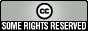# Calculus MATLAB Files

Many of the MATLAB scripts and functions here were originally developed to support an undergraduate course in "applied" calculus. Make sure you download both the .p and .m files. You can use the function or script with only the .p file, but you'll need the .m file for the associated help. For example, if you download RiemannSumsF.p you can use the RiemannSumsF function, provided you know how to use it. To read how to use it, however, you'll need RiemannSumsF.m. Once you've downloaded RiemannSumsF.m, you can enter help RiemannSumsF in the command window to read instructions on how to use the RiemannSumsF function.

 Files Description function2LaTeXstring.m, function2LaTeXstring.p Function: This function takes its function handle input, and if the function handle is an anonymous function (i.e., a formula as shown above), the dots before arithmetic operations (that vectorize the operations) and the @ are removed, parentheses () enclosing exponents are converted to braces {}, and * for multiplication is converted to \cdot. The resulting returned value of f_of_x is a string suitable for LaTeX formatting of objects in Figure Windows (e.g., titles). One limitation is that named functions (e.g., sin, cos, log, etc. are not converted to LaTeX format.) So, for example, cos is not converted to \cos and log is not converted to \ln, etc. limitDemo.m, limitDemo.p Script: Used to demonstrate the concept of limit by estimating the limit of a user-supplied function as x approaches some value a, which is also supplied by the user. limx-->a f(x) The user must supply the x-values taken closer and closer to a. The demonstration produces a graph showing the estimates and produces a table. Prerequisite: function2LaTeXstring limitDemoF.m, limitDemoF.p Function: Used to demonstrate the concept of limit by estimating the limit of a user-supplied function as x approaches some value a, which is also supplied by the user. limx-->a f(x) Prerequisite: function2LaTeXstring limitDemoF2.m, limitDemoF2.p Function: Another function used to demonstrate the concept of limit by estimating the limit of a user-supplied function as x approaches some value a, which is also supplied by the user. limx-->a f(x) Prerequisite: function2LaTeXstring limitDemoF3.m, limitDemoF3.p Function: Yet another function used to demonstrate the concept of limit by estimating the limit of a user-supplied function as x approaches some value a, which is also supplied by the user. limx-->a f(x) Prerequisite: function2LaTeXstring RiemannSumsF.m, RiemannSumsF.p Function: Used to demonstrates Riemann sums (left, midpoint, right, lower, or upper, depending on user-input). A table of approximations and related graphs showing the rectangles under the curve are generated. tangentFromSecLineF.m, tangentFromSecLineF.p Function: Used to demonstrate that the limit of a secant line slope lim_{h -->0} (f(a+h) - f(a))/h produces the slope of the tangent line to y=f(x) at x=a. A sequence of graphs of secant lines is generated in a manner such that the secant lines approach the tangent line. Prerequisite: function2LaTeXstring